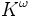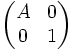# Stable general linear group over a field

Let$K$ be a field. The stable general linear group over$K$, denoted$GL(K)$, is defined in the following equivalent ways:
• Define$K^\omega$ as the direct sum of countably many copies of$K$.$GL(K)$ is defined as the group of those linear automorphisms of$K^\omega$ that fix pointwise all but finitely many of the copies.
• Consider the general linear groups$GL_n(K)$, with a natural inclusion map$GL_n(K) \to GL_{n+1}(K)$ that sends$A$ to the matrix with block description$\begin{pmatrix}A & 0 \\ 0 & 1 \\\end{pmatrix}$.$GL(K)$ is defined as the direct limit of this sequence of groups with homomorphisms.